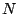Next: Automation and voice management Up: Audio and control computations Previous: MIDI-style synthesizer   Contents   Index

# Exercises

1. How many partials of a tone at A 440 can be represented digitally at a sample rate of 44100 Hertz?

2. What frequency would you hear if you synthesized a sinusoid at 88000 Hertz at a sample rate of 44100?

3. Suppose you are synthesizing sound at 44100 Hertz, and are computing 64-sample audio blocks. A control event is scheduled to happen at an elapsed time of exactly one second, using the fast-as-possible update scheme. At what sample does the update actually occur?

4. Sampling at 44100, we wish to approximately play a tone at middle C by repeating a fixed waveform every N samples. What value ofshould we choose, and how many cents (Page) are we off from the ``true" middle C?

5. Two sawtooth waves, of unit amplitude, have frequencies 200 and 300 Hertz, respectively. What is the periodicity of the sum of the two? What if you then wrapped the sum back to the range from 0 to 1? Does this result change depending on the relative phase of the two?

6. Two sawtooth waves, of equal frequency and amplitude and one half cycle out of phase, are summed. What is the waveform of the sum, and what are its amplitude and frequency?

7. What is the relative level, in decibels, of a sawtooth wave's third harmonic (three times the fundamental) compared to that of the fundamental?

8. Suppose you synthesize a 44000-Hertz sawtooth wave at a sample rate of 44100 Hertz. What is the resulting waveform?

9. Using the techniques of Section 3.7, draw a block diagram for generating two phase-locked sinusoids at 500 and 700 Hertz.

10. Draw a block diagram showing how to use thresholding to detect when one audio signal exceeds another one in value. (You might want to do this to detect and filter out feedback from speakers to microphones.)Next: Automation and voice management Up: Audio and control computations Previous: MIDI-style synthesizer   Contents   Index
Miller Puckette 2006-12-30